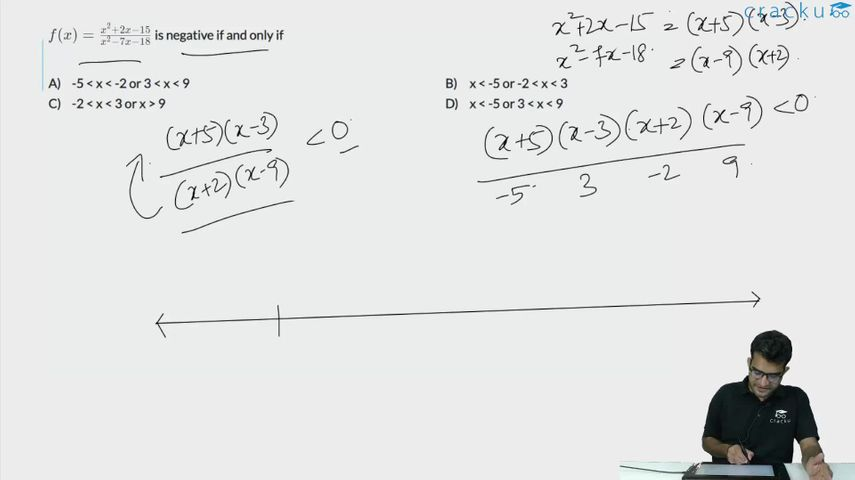Question 15

# $$f(x) = \frac{x^2 + 2x - 15}{x^2 - 7x - 18}$$ is negative if and only if

Solution

$$f(x) = \frac{x^2 + 2x - 15}{x^2 - 7x - 18}$$<0

$$\frac{\left(x+5\right)\left(x-3\right)}{\left(x-9\right)\left(x+2\right)}<0$$

We have four inflection points -5, -2, 3, and 9.

For x<-5, all four terms (x+5), (x-3), (x-9), (x+2) will be negative. Hence, the overall expression will be positive. Similarly, when x>9, all four terms will be positive.

When x belongs to (-2,3), two terms are negative and two are positive. Hence, the overall expression is positive again.

We are left with the range (-5,-2) and (3,9) where the expression will be negative.

### View Video Solution